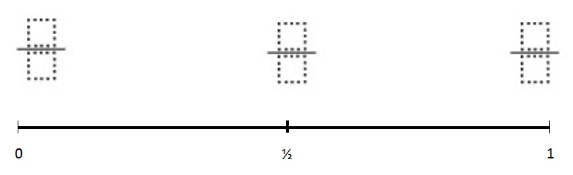Home > Grade 4 > Benchmark Fractions

# Benchmark Fractions

Directions: Use the digits 1 to 9, no more than once, to create three fractions that are as close to zero, one half and one as possible. NOTE: Close as possible is measured by adding up all the differences and making it the least possible value.### Hint

What do we know about fractions that are equivalent to one? How could you make a fraction that equals close to one?

Multiple solutions

1/7, 3/6, 8/9
The total difference: 16/63

Source: Darbie Valenti

## Multiplying Two-Digit Numbers – Closest to 7,000

Directions: Directions: Using the digits 1 to 9 at most one time each, fill in …

1.Would a better answer not be:

1/9, 2/4 (or 3/6), and 7/8

Giving a total difference of 1/9 + 1/8 = 17/72 < 16/63

•James…That is the answer I got as well.

2.Just a thought – given that it says use the digits 1 to 9, I would assume you could use multi-digit numbers.
3/76, 4/8 and 21/19…

I didn’t do the fractions, but the difference is about a tenth less than the previous answer

•Cheryl Canterbury

Perhaps, but you used the digit 1 more than once.

3.4.5.Scarlett Salaz

1/10,3/6 and 2/3!

6.1/9

3/6

4/5